# Buses

At the bus stop is at 10 o'clock met buses No. 2 and No. 9. Bus number 2 runs at an interval of 4 minutes and the bus number 9 at intervals of 9 minutes. How many times the bus meet to 18:00 local time?

Result

n =  13

#### Solution:

Leave us a comment of this math problem and its solution (i.e. if it is still somewhat unclear...):Be the first to comment!#### Following knowledge from mathematics are needed to solve this word math problem:

Do you want to calculate least common multiple two or more numbers?

## Next similar math problems:

1. Buses 4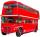intervals: 1st bus 40 min. 2nd bus 2h 3rd bud 20min How long take them to meet - as soon as possible?
2. Tram lines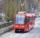Trams of five lines driven at intervals of 5,8,10,12 and 15 minutes. At 12 o'clock come out of the station at the same time. About how many hours again all meet? How many times have earch tram pass for this stop?
3. Trams 2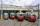Square passes two lines of tram. One running every nine minutes, a second interval of 15 minutes. Exactly at 12 o'clock arrived two tram lines in the square. How soon should a similar situation arise again?Added together and write as decimal number: LXVII + MLXIV
5. LCMCommon multiple of three numbers is 3276. One number is in this number 63 times, second 7 times, third 9 times. What are the numbers?
6. LCM of two numberFind the smallest multiple of 63 and 147
7. The smallest numberWhat is the smallest number that can be divided by both 5 and 7
8. Primes 2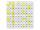Which prime numbers is number 2025 divisible?
9. NumberDetermine unknown number if you know that difference between five times and triple of number is 42.
10. BiketrialKamil was biketrial. Before hill he set the forward gear with 42 teeth and the back with 35 teeth. After how many exercises (rotation) of the front wheel both wheels reach the same position?
11. Apples 2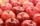How many minimum apples are in the cart, if possible is completely divided into packages of 6, 14 and 21 apples?
12. Dance ensemble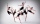The dance ensemble took the stage in pairs. During dancing, the dancers gradually formed groups of four, six and nine. How many dancers have an ensemble?
13. Lcm simpleFind least common multiple of this two numbers: 140 175.
14. Balls groups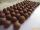Karel pulled the balls out of his pocket and divide it into the groups. He could divide them in four, six or seven, and no ball ever left. How little could be a ball?
15. Hr to minSue biked to school in 5/12 of an hour. How many minutes did it take her to ride to school?
16. Minutes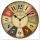Determine the difference in minutes: T1 = 2 3/20 h T2 = 2.3 h
17. Father and son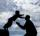Father is 44 years old, his son 16 years. Determine how many years ago was the father five times older than the son.